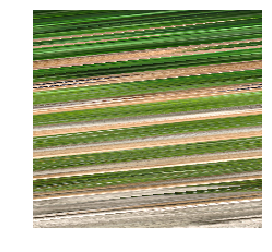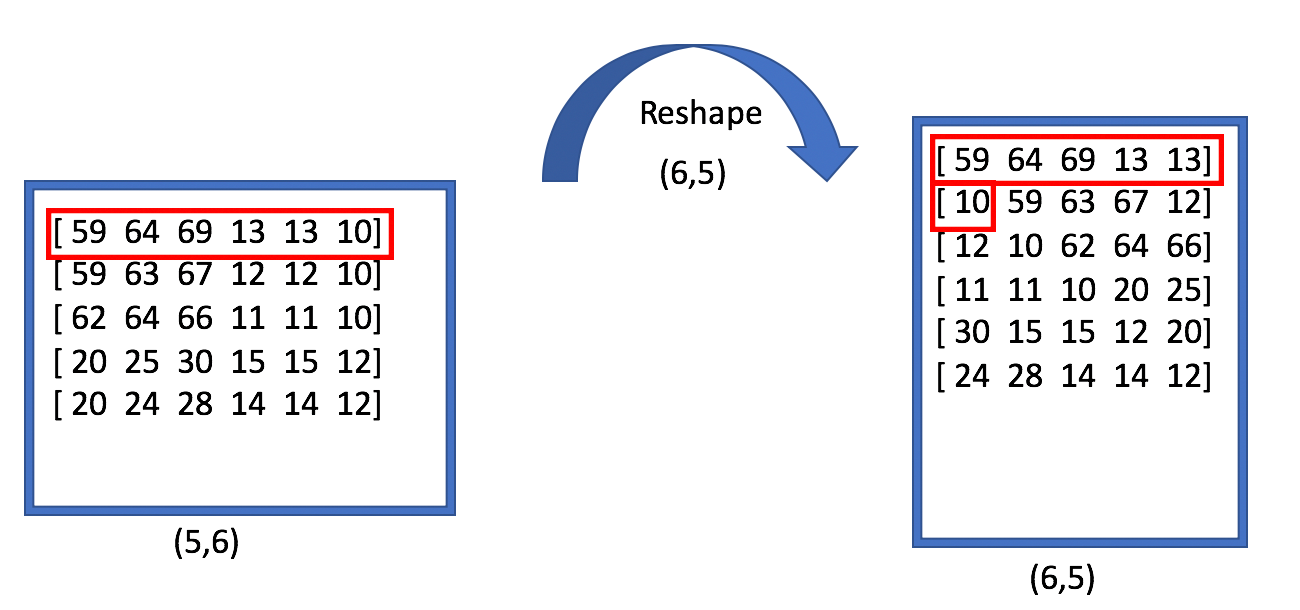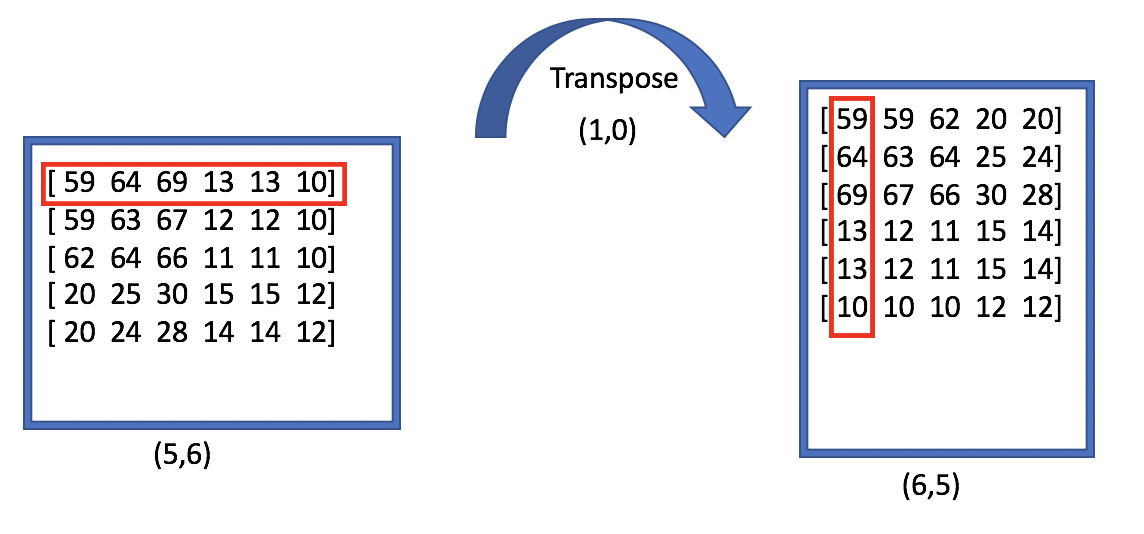# Difference between reshape and transpose operators¶

What does it mean if MXNet gives you an error like the this?

Check failed: shape_.Size() == shape.Size() (127872 vs. 25088) NDArray.Reshape: target shape must have the same size as current shape when recording with autograd.


This error message tells you that the data being passed to your model or between layers in the model is not in the correct format. Modifying the shape of tensors is a very common operation in Deep Learning. For instance, when using pretrained neural networks it is often necessary to adjust the input data dimensions to correspond to what the network has been trained on, e.g. tensors of shape [batch_size, channels, width, height]. This notebook discusses briefly the difference between the operators Reshape and Transpose. Both allow you to change the shape, however they are not the same and are commonly mistaken.

import matplotlib.pyplot as plt
import matplotlib.image as mpimg
import mxnet as mx
from mxnet import gluon
import numpy as np

img_array = mpimg.imread('https://raw.githubusercontent.com/dmlc/web-data/master/mxnet/doc/tutorials/basic/transpose_reshape/cat.png')
plt.imshow(img_array)
plt.axis("off")
print (img_array.shape)


(157, 210, 3)The color image has the following properties:

• width: 210 pixels
• height: 157 pixels
• colors: 3 (RGB)

Now let’s reshape the image in order to exchange width and height dimensions.

reshaped = img_array.reshape((210,157,3))
print (reshaped.shape)
plt.imshow(reshaped)
plt.axis("off")


(210,157,3)As we can see the first and second dimensions have changed. However the image can’t be identified as cat any longer. In order to understand what happened, let’s have a look at the image below.While the number of rows and columns changed, the layout of the underlying data did not. The pixel values that have been in one row are still in one row. This means for instance that pixel 10 in the upper right corner ends up in the middle of the image instead of the lower left corner. Consequently contextual information gets lost, because the relative position of pixel values is not the same anymore. As one can imagine a neural network would not be able to classify such an image as cat.

Transpose instead changes the layout of the underlying data.

transposed = img_array.transpose((1,0,2))
plt.imshow(transposed)
plt.axis("off")As we can see width and height changed, by rotating pixel values by 90 degrees. Transpose does the following:As shown in the diagram, the axes have been flipped: pixel values that were in the first row are now in the first column.

# When to transpose/reshape with MXNet¶

In this chapter we discuss when transpose and reshape is used in MXNet.

## Channel first for images¶

Images are usually stored in the format height, wight, channel. When working with convolutional layers, MXNet expects the layout to be NCHW (batch, channel, height, width). This is in contrast to Tensorflow, where image tensors are in the form NHWC. MXNet uses NCHW layout because of performance reasons on the GPU. When preprocessing the input images, you may have a function like the following:

def transform(data, label):
return data.astype(np.float32).transpose((2,0,1))/255.0, np.float32(label)


Images may also be stored as 1 dimensional vector for example in byte packed datasets. For instance, instead of [28,28,1] you may have [784,1]. In this situation you need to perform a reshape e.g. ndarray.reshape((1,28,28))

## TNC layout for RNN¶

When working with LSTM or GRU layers, the default layout for input and ouput tensors has to be TNC (sequence length, batch size, and feature dimensions). For instance in the following network the input goes into a 1 dimensional convolution layer and whose output goes into a GRU cell. Here the tensors would mismatch, because Conv1D takes data as NCT, but GRU expects it to be NTC. To ensure that the forward pass does not crash, we need to do a tensor transpose. We can do this by defining a HybridLambda.

network = gluon.nn.HybridSequential()
with network.name_scope():
network.add(gluon.nn.HybridLambda(lambda F, x: F.transpose(x, (0, 2, 1))))

network.hybridize()
network.initialize(mx.init.Xavier(), ctx=mx.cpu())
a = mx.random.uniform(shape=(1,100,1000))
network(a)
output = network(a)
print (output.shape)


(1, 999, 128)

## Advanced reshaping with MXNet ndarrays¶

It is sometimes useful to automatically infer the shape of tensors. Especially when you deal with very deep neural networks, it may not always be clear what the shape of a tensor is after a specific layer. For instance you may want the tensor to be two-dimensional where one dimension is the known batch_size. With mx.nd.array(-1, batch_size) the first dimension will be automatically inferred. Here is a simplified example:

batch_size = 100
input_data = mx.random.uniform(shape=(batch_size, 20,100))
reshaped = input_data.reshape(batch_size, -1)
print (input_data.shape, reshaped.shape)


(100L, 20L, 100L), (100L, 2000L)

The reshape function of MXNet’s NDArray API allows even more advanced transformations: For instance:0 copies the dimension from the input to the output shape, -2 copies all/remainder of the input dimensions to the output shape. With -3 reshape uses the product of two consecutive dimensions of the input shape as the output dim. With -4 reshape splits one dimension of the input into two dimensions passed subsequent to -4. Here an example:

x = mx.nd.random.uniform(shape=(1, 3, 4, 64, 64))


Assume x with the shape [batch_size, channel, upscale, width, height] is the output of a model for image superresolution. Now we want to apply the upscale on width and height, to increase the 64x64 to an 128x128 image. To do so, we can use advanced reshaping, where we have to split the third dimension (upscale) and multiply it with width and height. We can do

x = x.reshape(1, 3, -4, 2, 2, 0, 0)
print (x.shape)


(1L, 3L, 2L, 2L, 64L, 64L)

This splits up the third dimension into [2,2], so (1L, 3L, 4L , 64L, 64L) becomes (1L, 3L, 2L , 2L , 64L, 64L) The other dimensions remain unchanged. In order to multiply the new dimensions with width and height, we can do a transpose and then use reshape with -3.

x = x.transpose((0, 1, 4, 2, 5, 3))
print (x.shape)
x = x.reshape(0, 0, -3, -3)
print (x.shape)


(1L, 3L, 64L, 2L, 64L, 2L)

(1L, 3L, 128L, 128L)

Reshape -3 will calculate the dot product between the current and subsequent column. So (1L, 3L, 64L , 2L , 64L, 2L ) becomes (1L, 3L, 128L , 128L )

## Most Common Pitfalls¶

In this section we want to show some of the most common pitfalls that happen when your input data is not correctly shaped.

### Forward Pass¶

You execute the forward pass and get an error message followed by a very long stacktrace, for instance:

*** Error in python': free(): invalid pointer: 0x00007fde5405a918 ***
======= Backtrace: =========
/lib/x86_64-linux-gnu/libc.so.6(+0x777e5)[0x7fdf475927e5]
/lib/x86_64-linux-gnu/libc.so.6(+0x8037a)[0x7fdf4759b37a]
/lib/x86_64-linux-gnu/libc.so.6(cfree+0x4c)[0x7fdf4759f53c]
/home/ubuntu/anaconda3/lib/python3.6/site-packages/mxnet/libmxnet.so(MXExecutorReshape+0x1852)[0x7fdecef2c6e2]
python(_PyObject_FastCallDict+0x8b)[0x56457eba2d7b]
python(+0x19e7ce)[0x56457ec327ce]
python(_PyEval_EvalFrameDefault+0x2fa)[0x56457ec54cba]
python(+0x197dae)[0x56457ec2bdae]
[...]


This happens when you your data does not have the shape [batch_size, channel, width, height] e.g. your data may be a one-dimensional vector or when the color channel may be the last dimension instead of the second one.

### Backward Pass¶

In other cases the forward pass may not fail, but the shape of the network output is not as expected. For instance in our previous RNN example, nothing is preventing us to skip the transpose.

network = gluon.nn.HybridSequential()
with network.name_scope():
#network.add(gluon.nn.HybridLambda(lambda F, x: F.transpose(x, (0, 2, 1))))

network.hybridize()
network.initialize(mx.init.Xavier(), ctx=mx.cpu())
a = mx.random.uniform(shape=(1,100,1000))
output = network(a)
print (output.shape)


(1, 196, 128)

Instead of (1, 999, 128) the shape is now (1, 196, 128). But during the training loop, calculating the loss would crash because of shape mismatch between labels and output. You may get an error like the following:

mxnet.base.MXNetError: [10:56:29] src/ndarray/ndarray.cc:229: Check failed: shape_.Size() == shape.Size() (127872 vs. 25088) NDArray.Reshape: target shape must have the same size as current shape when recording with autograd.

Stack trace returned 6 entries:
[bt] (0) 0   libmxnet.so                         0x00000001126c0b90 libmxnet.so + 15248
[bt] (1) 1   libmxnet.so                         0x00000001126c093f libmxnet.so + 14655
[bt] (2) 2   libmxnet.so                         0x0000000113cd236d MXNDListFree + 1407789
[bt] (3) 3   libmxnet.so                         0x0000000113b345ca MXNDArrayReshape64 + 970
[bt] (4) 4   libffi.6.dylib                      0x000000010b399884 ffi_call_unix64 + 76
[bt] (5) 5   ???                                 0x00007fff54cadf50 0x0 + 140734615969616
`